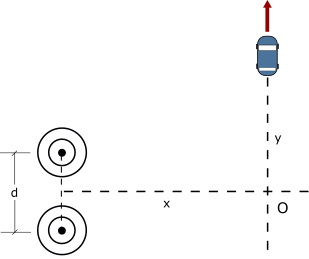# Two radio antennas separated by d = 307 m, as shown in the figure below, simultaneously broadcast...

## Question:

Two radio antennas separated by d = 307 m, as shown in the figure below, simultaneously broadcast identical signals at the same wavelength. A car travels due north along a straight line at position x = 1,200 m from the center point between the antennas, and its radio receives the signal. Hint: Do not use the small-angle approximation in this problem.

a.) If the car is at the position of the third maximum after that at point O when it has traveled a distance of y = 400 m northward, what is the wavelength of the signals?

b.) How much farther must the car travel to encounter the next minimum signal?## Interference of Waves:

If two coherent sources of waves of the same wavelength emit waves at the same time, the combined wave resulting from the two wave sources will form the pattern of minima and maxima called an interference pattern. At any point in space where two waves are in phase, the intensity increases and we observe a maximum. If two waves are out of phase, the minimum is observed since amplitudes cancel each other out.

a) The condition for the constructive interference can be written as follows:

{eq}\sqrt{x^2 + \left ( y + \dfrac {d}{2} \right )^2} - \sqrt{x^2 + \left ( y - \dfrac {d}{2} \right )^2} = m \lambda {/eq}

Here

• {eq}x = 1200 \ m {/eq} is the distance from the antennas to the line of car motion;
• {eq}y = 400 \ m {/eq} is the distance car traveled along y-direction;
• {eq}d = 307 \ m {/eq} is the separation between antennas;
• {eq}m = 3 {/eq} is the order of the maximum the car encounters;

Computing the wavelength, we obtain:

{eq}\lambda = \dfrac {\sqrt{(1200 \ m)^2 + \left ( 400 \ m + \dfrac {307 \ m}{2} \right )^2} - \sqrt{(1200 \ m)^2 + \left ( 400 \ m - \dfrac {307 \ m}{2} \right )^2}}{3} \approx \boxed {\color{blue}{32.1 \ m}} {/eq}

b) The next minimum would correspond to the path-length difference that is equal to {eq}\dfrac {7}{2}\lambda {/eq}

Solving for the y-position, we obtain after some tedious but simple algebra:

{eq}4y^2 (d^2 - 4 \lambda^2) + 15 \lambda^2 d y - \lambda^2 \left [ 16 d^2 + 64 x^2 - \dfrac {2401}{16}\lambda^2 \right ] = 0 {/eq}

Solving for the y, we come up with the following expression:

{eq}y = \dfrac {-15\lambda^2d + \sqrt{9604\lambda^6 + 1024 x^2d^2 \lambda^2 - 4096 x^2\lambda^4 - 3200 \lambda^4 d^2 + 256 \lambda^2 d^4}}{8(d^2 - 4\lambda^2)} {/eq}

Computation yields:

{eq}y = \dfrac {-15\cdot (32.1 \ m)^2 \cdot 307 \ m + \sqrt{9604 \cdot (32.1 \ m)^6 + 1024 \cdot (1200 \ m)^2 \cdot (307 \ m)^2 \cdot (32.1 \ m)^2 - 4096 \cdot (1200 \ m)^2 \cdot (32.1 \ m)^4 - 3200 \cdot (32.1 \ m)^4 \cdot (307 \ m)^2 + 256 \cdot (32.1 \ m)^2 \cdot (307 \ m)^4}}{8((307 \ m)^2 - 4 \cdot (32.1 \ m)^2)} \approx 511 \ m {/eq}

Therefore, the car must travel approximately

{eq}\Delta y = 511 \ m - 400 \ m = \boxed {\color{red}{111 \ m}} {/eq}